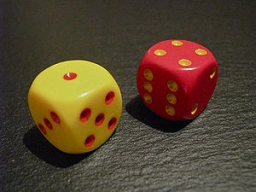# Probability 81591

We roll the dice three times. Calculate the probability of getting an even number on the first, second, or third toss.

p =  7/8

### Step-by-step explanation:Did you find an error or inaccuracy? Feel free to write us. Thank you!

Tips for related online calculators
Need help calculating sum, simplifying, or multiplying fractions? Try our fraction calculator.
Would you like to compute the count of combinations?